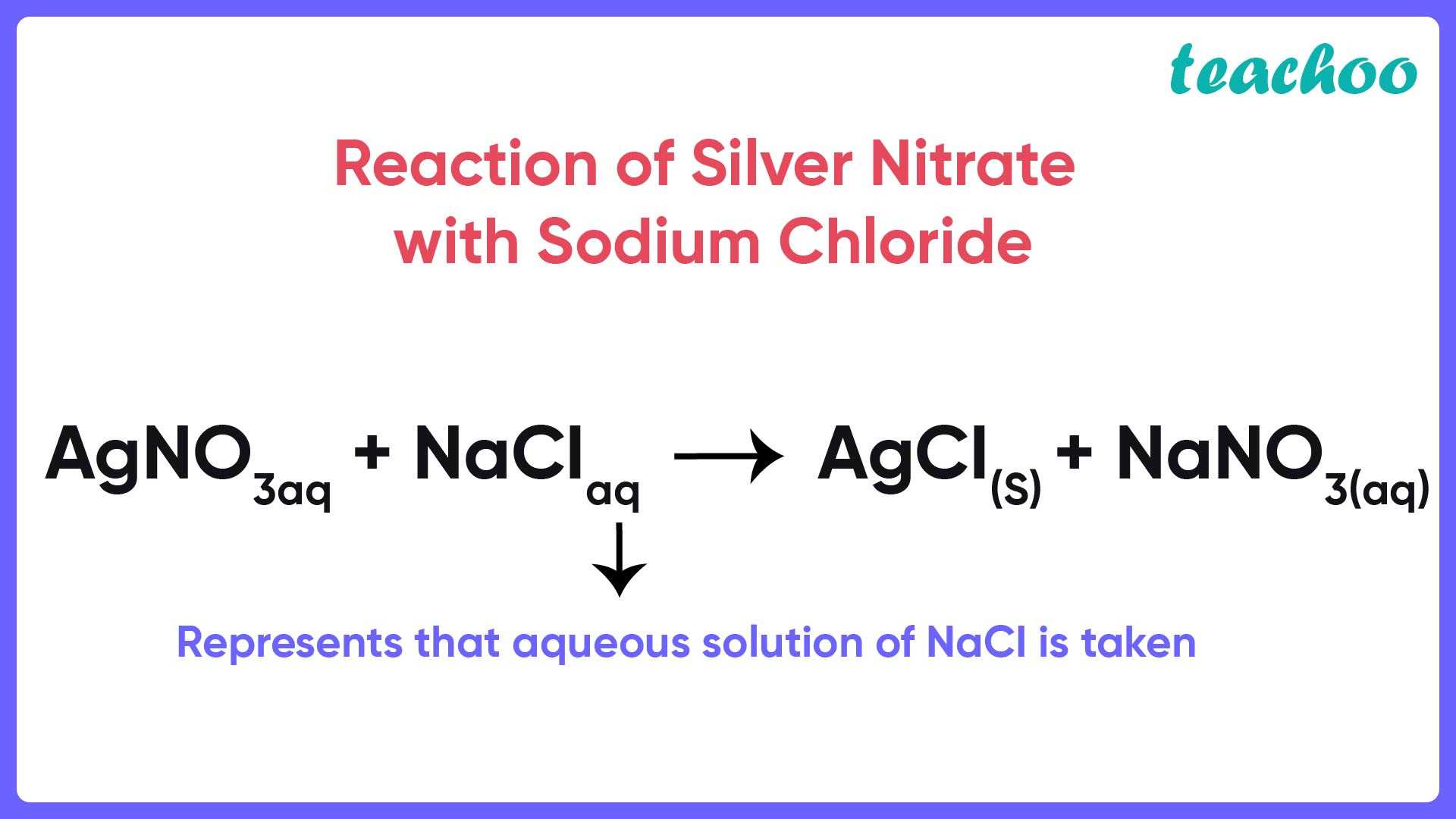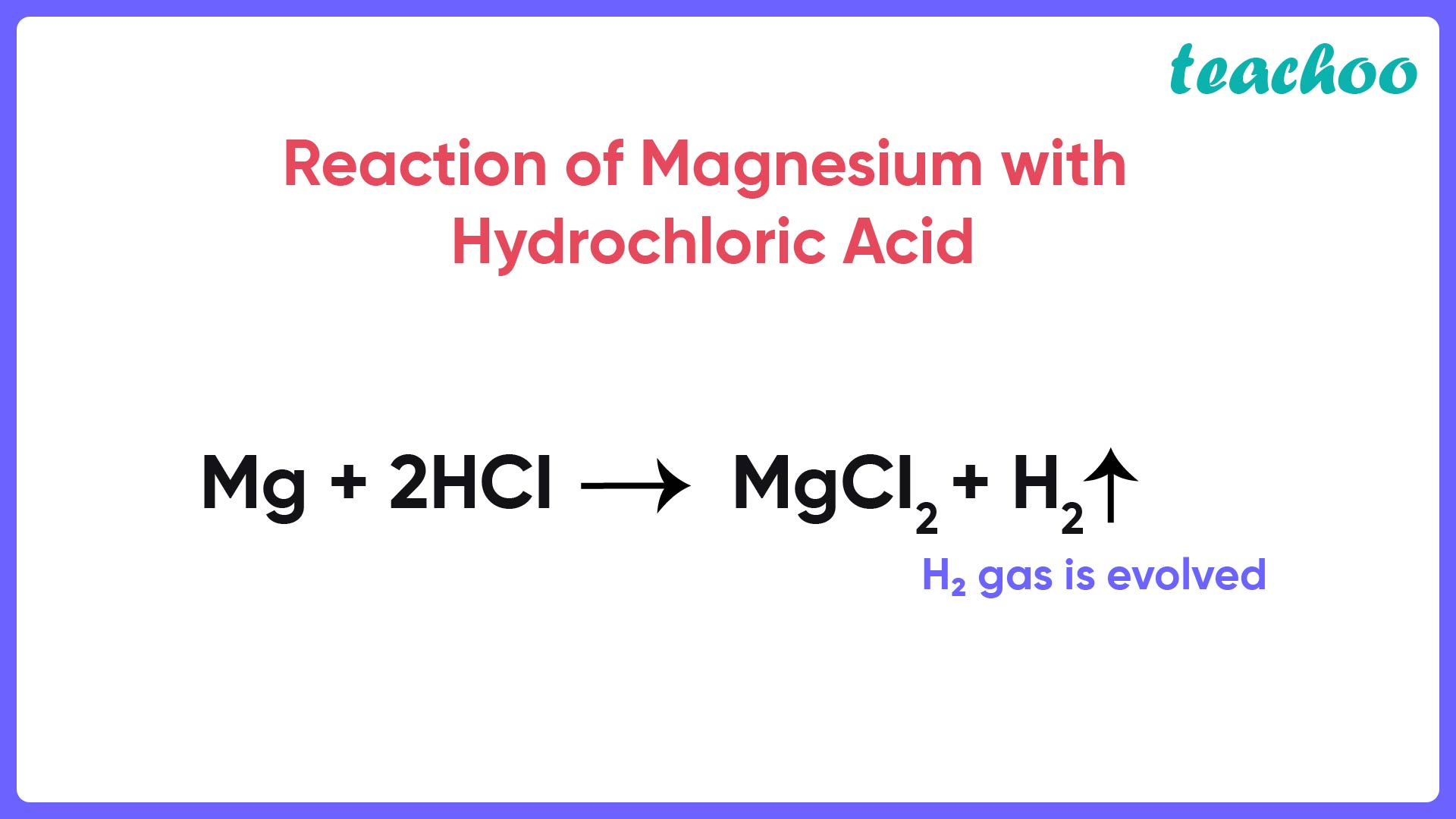Case Based Questions (MCQ)

Class 10
Chapter 1 Class 10 - Chemical Reactions and Equations

## (iv) ↓

Ans.So, the correct a nswer is (iii) (aq)

## (iv) (g)

Ans.So, the correct a nswer is (iii) ↑

## (iv) (g) and (aq)

Ans. Here,

• Since Na is reacting with water , the NaOH produced will be in aqueous medium , hence ( aq) symbol will be used.
• H 2 is a gas which is evolved during the reaction, so we use the symbol (g) for it.

So, the correct answer is (i) (aq) and (g)

## (iv) 2NaCl + 2H 2 O → NaOH + Cl 2 + H 2

Ans.

Explanation:

A balanced chemical equation is one in which the number of atoms of both reactants and products are equal.

In reaction I:

 Na H O Cl Reactant 1 4 2 1 Product 2 4 2 4

Since the number of Na and Cl atoms in reactant and product is not equal, it is not a balanced equation.

In reaction II:

 Na H O Cl Reactant 2 2 1 2 Product 2 4 2 4

Since the number of H, O and Cl atoms in reactant and product is not equal. It is not a balanced equation.

In reaction III:

 Na H O Cl Reactant 2 4 2 2 Product 2 4 2 2

Since the number of all atoms in reactants and products is equal, it is a balanced equation.

In Reaction IV:

 Na H O Cl Reactant 2 4 2 2 Product 1 3 1 2

Since the number of Na, H, and O atoms is not equal in reactant and product, it is not a balanced equation.

So, the correct answer is  (iii) 2NaCl + 2H 2 O → 2NaOH + Cl 2 + H 2

## (iv) Mg (s) + H 2 SO 4 (l) → MgSO 4 (s) + H 2

Ans. (ii) Mg (s) + H 2 SO 4 (aq) → MgSO 4 (aq) + H 2

Explanation:

A balanced chemical equation is one in which the number of atoms of both reactants and products are equal.

In reaction I:

 Mg H O S Reactant 1 2 2 1 Product 1 2 4

In reaction II:

 Mg H O S Reactant 1 2 4 1 Product 1 2 4 1

Since the number of all atoms in reactants and products is equal, it is a balanced equation.

In reaction III:

 Mg H O S Reactant Product

In Reaction IV:

 Mg H O S Reactant Product

Learn in your speed, with individual attention - Teachoo Maths 1-on-1 Class